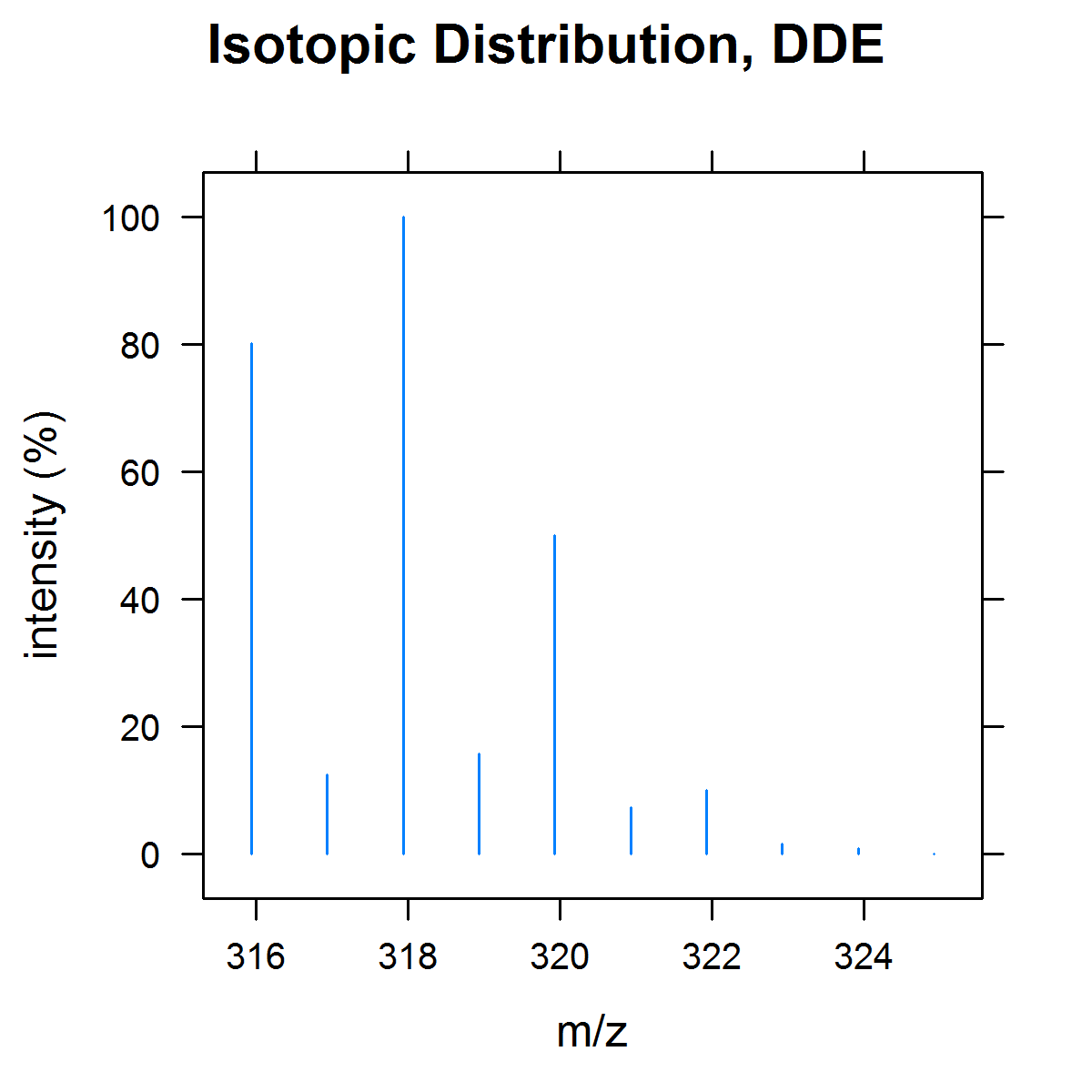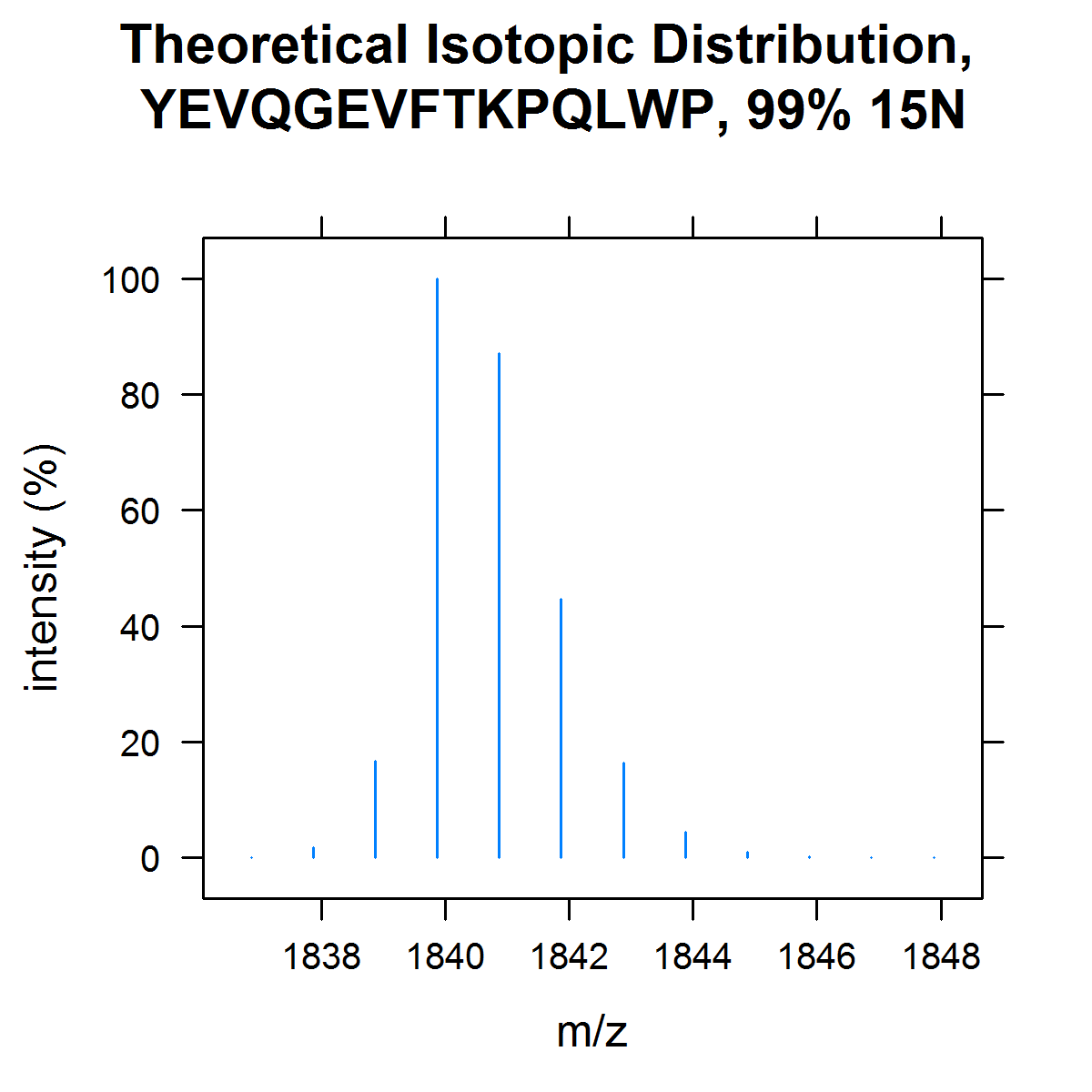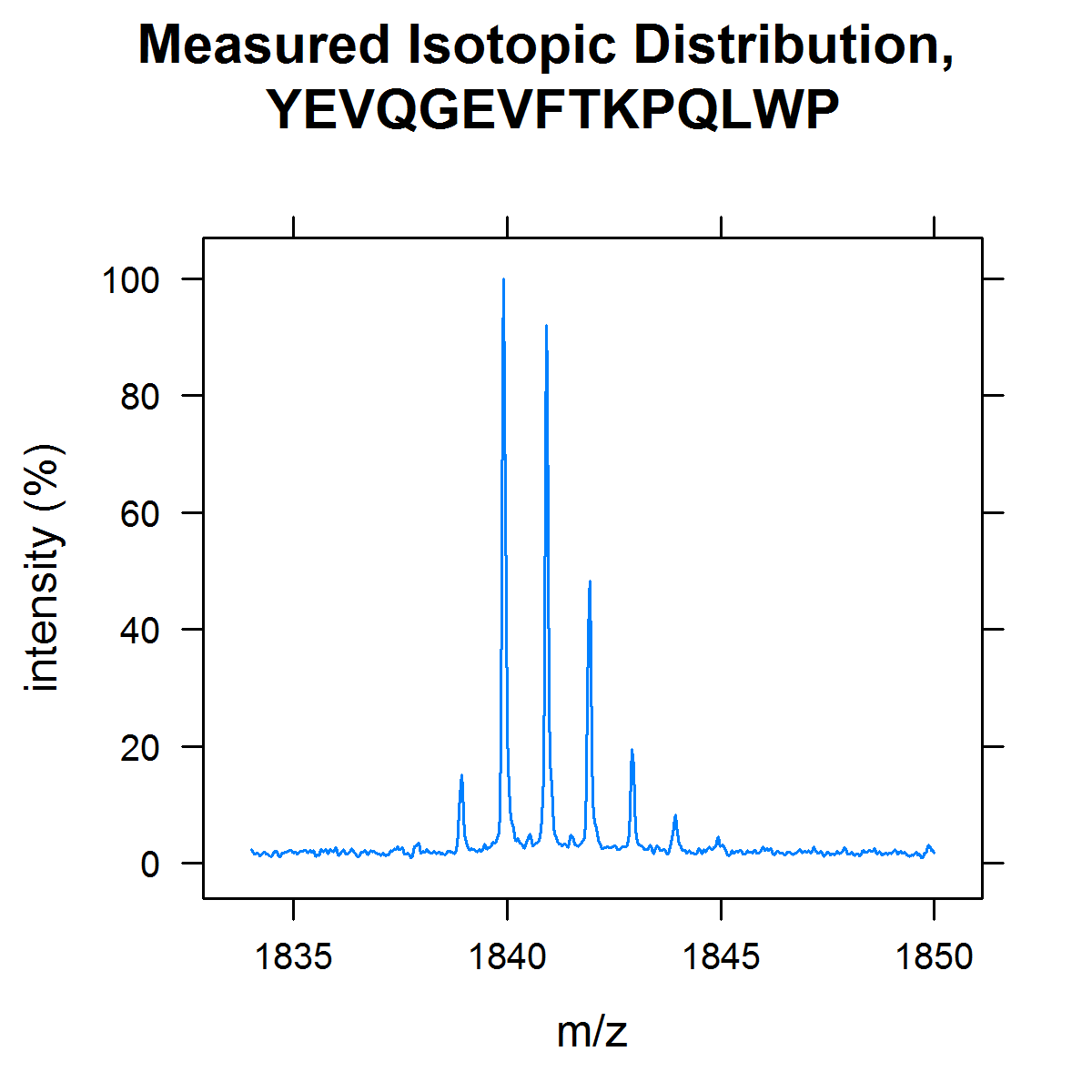# Vignette for OrgMassSpecR: Examples

### Introduction

`OrgMassSpecR` is a package for organic/biological mass spectrometry. This vignette demonstrates some of the functions in a larger context than the help file examples.

First, load the package.

``````library(OrgMassSpecR)
``````
``````Loading required package: grid
``````

### Small Molecules

The functions `MonoisotopicMass` and `IsotopicDistribution` assist in identifying unknown mass spectra, or in confirming peak identities in known spectra. The monoisotopic mass of DDE, a breakdown product of the pesticide DDT, is calculated as follows.

``````MonoisotopicMass(formula = list(C=14, H=8, Cl=4))
``````
`````` 315.938
``````

The monoisotopic masses due to successive losses of chlorine, which are observed in the electron impact mass spectrum of DDE, are calculated by repeated calls to `MonoisotopicMass`.

``````MonoisotopicMass(formula = list(C=14, H=8, Cl=3))
``````
`````` 280.9692
``````
``````MonoisotopicMass(formula = list(C=14, H=8, Cl=2))
``````
`````` 246.0003
``````

The monoisotopic mass of 13C12 labeled DDE (an internal/surrogate standard for the quantification of DDE) is calculated using the list component `x`.

``````MonoisotopicMass(formula = list(C=2, H=8, Cl=4, x = 12),
isotopes = list(x = 13.0033548378))
``````
`````` 327.9783
``````

The isotopic distribution of DDE is simulated using `IsotopicDistribution`. This function uses a binning approach based on `sample`, where the probabilities are the natural abundances of the isotopes. The output of this function is a table, but it is often helpful to plot the distribution.

``````dde.dist <- IsotopicDistribution(formula = list(C=14, H=8, Cl=4))
dde.dist
``````
``````       mz intensity percent
1  315.94      2879   80.19
2  316.94       448   12.48
3  317.94      3590  100.00
4  318.94       567   15.79
5  319.93      1799   50.11
6  320.94       265    7.38
7  321.93       358    9.97
8  322.93        59    1.64
9  323.93        32    0.89
10 324.93         3    0.08
``````
``````# plot
library(lattice)
print(xyplot(percent ~ mz,
data = dde.dist,
type = "h",
xlab = "m/z",
ylab = "intensity (%)",
main = "Isotopic Distribution, DDE")
)
``````The similarity between two mass spectra can be examined using `SpectrumSimilarity`. This function makes a head-to-tail plot of the spectra and calculates a mass spectral similarity score based on the dot product of the two mass-aligned intensity vectors. See the help file for an example.

### Proteins and Peptides

The following functions assist in setting up multiple reaction monitoring (MRM) assays for the quantification of proteins. These assays require the selection of “signature peptides” (1,2).

Peptides resulting from a protein digestion with trypsin or pepsin can be prediced using `Digest`.

``````hsa <- Digest(example.sequence)
head(hsa)
``````
``````                peptide start stop mc      mz1      mz2     mz3
1                  DAHK     1    4  0  470.236  235.622 157.417
2                SEVAHR     5   10  0  698.358  349.683 233.458
3                    FK    11   12  0  294.181  147.594  98.732
4              DLGEENFK    13   20  0  951.442  476.225 317.819
5 ALVLIAFAQYLQQCPFEDHVK    21   41  0 2490.285 1245.646 830.767
6            LVNEVTEFAK    42   51  0 1149.615  575.311 383.877
``````

Next, peptides between 5 and 12 amino acids are selected (the range is somewhat arbitrary; small peptides may not be specific to the target protein, large peptides may have low sensitivity).

``````hsa.sub <- subset(hsa, nchar(hsa\$peptide) >= 5 & nchar(hsa\$peptide) <= 12)
head(hsa.sub)
``````
``````        peptide start stop mc      mz1     mz2     mz3
2        SEVAHR     5   10  0  698.358 349.683 233.458
4      DLGEENFK    13   20  0  951.442 476.225 317.819
6    LVNEVTEFAK    42   51  0 1149.615 575.311 383.877
8     SLHTLFGDK    65   73  0 1017.536 509.272 339.850
9      LCTVATLR    74   81  0  933.519 467.263 311.844
10 ETYGEMADCCAK    82   93  0 1434.533 717.770 478.849
``````

The filtered table can be used to screen a digest for the presence of these peptides by operating the triple quadrupole instrument in Q1 selected ion mode with Q2 and Q3 open. Assuming peptides YLYEIAR and AEFAEVSK are found (the number is kept small for this example), the next step is to determine their most intense MRM transitions. The b- and y-ions of the peptides are calculated using `FragmentPeptide`.

``````transitions <- FragmentPeptide(c("YLYEIAR", "AEFAEVSK"))
head(transitions)
``````
``````   ms1seq   ms1z1  ms1z2   ms1z3 ms2seq ms2type   ms2mz
1 YLYEIAR 927.493 464.25 309.836      Y  [b1]1+ 164.071
2 YLYEIAR 927.493 464.25 309.836     YL  [b2]1+ 277.155
3 YLYEIAR 927.493 464.25 309.836    YLY  [b3]1+ 440.218
4 YLYEIAR 927.493 464.25 309.836   YLYE  [b4]1+ 569.261
5 YLYEIAR 927.493 464.25 309.836  YLYEI  [b5]1+ 682.345
6 YLYEIAR 927.493 464.25 309.836 YLYEIA  [b6]1+ 753.382
``````

This table can be used to screen the MRM transitions. The table is formatted to facilitate easy selection of the appropriate precursor ion and product ion charge states.

Note: both `Digest` and `FragmentPepetide` by default use `IAA=TRUE`, specifying iodoacetylated cysteine.

Once the signature peptides for the target protein have been determined, MRM transitions for the internal standard peptides must be set up. Generally, either synthetic 13C-labeled peptides or 15N-labeled proteins are used. 15N-labeled proteins are added prior to the digestion to yield 15N-labeled peptides.

The MRM transitions for YLYEIAR with the terminal arginine 13C-labeled are calculated as follows.

``````c13.labeled <- FragmentPeptide("YLYEIAr", custom = list(code = "r",
mass = MonoisotopicMass(formula = list(C=6, H=12, N=4, O=1),
isotopes = list(C=13.0033548378))))
head(c13.labeled)
``````
``````   ms1seq   ms1z1  ms1z2   ms1z3 ms2seq ms2type   ms2mz
1 YLYEIAr 933.514 467.26 311.843      Y  [b1]1+ 164.071
2 YLYEIAr 933.514 467.26 311.843     YL  [b2]1+ 277.155
3 YLYEIAr 933.514 467.26 311.843    YLY  [b3]1+ 440.218
4 YLYEIAr 933.514 467.26 311.843   YLYE  [b4]1+ 569.261
5 YLYEIAr 933.514 467.26 311.843  YLYEI  [b5]1+ 682.345
6 YLYEIAr 933.514 467.26 311.843 YLYEIA  [b6]1+ 753.382
``````

The MRM transitions for fully 15N-labeled YLYEIAR are calculated as follows. Note that `FragmentPeptide` and `Digest` do not label the nitrogens incorporated into the peptide due to iodoacetamide treatment (when IAA = TRUE and 15N = TRUE).

``````n15.labeled <- FragmentPeptide("YLYEIAR", N15 = TRUE)
head(n15.labeled)
``````
``````   ms1seq   ms1z1   ms1z2   ms1z3 ms2seq ms2type   ms2mz
1 YLYEIAR 937.464 469.236 313.159      Y  [b1]1+ 165.068
2 YLYEIAR 937.464 469.236 313.159     YL  [b2]1+ 279.149
3 YLYEIAR 937.464 469.236 313.159    YLY  [b3]1+ 443.209
4 YLYEIAR 937.464 469.236 313.159   YLYE  [b4]1+ 573.249
5 YLYEIAR 937.464 469.236 313.159  YLYEI  [b5]1+ 687.330
6 YLYEIAR 937.464 469.236 313.159 YLYEIA  [b6]1+ 759.364
``````

An acquired full-scan peptide spectrum can be plotted using `PeptideSpectrum`. The peptide sequence must be known to determine the fragment ion identities (i.e., the function does not sequence the peptide de novo). This function was created to catalog full-scan mass spectra and double check that the most intense ions observed by MRM screens correspond to the most intense ions observed in full scan mode. See the help file for an example.

Before use as an internal standard, the 15N incorporation in the expressed protein should be measured. The incorporation should be high enough that the isotopic envelop of the internal standard signature peptide does not overlap with that of the corresponding unlabeled signature peptide.

The isotopic distribution of 15N labeled peptides is calculated using `IsotopicDistributionN`.

``````theoretical.dist <- IsotopicDistributionN("YEVQGEVFTKPQLWP", incorp = 0.99)
print(xyplot(percent ~ mz,
data = theoretical.dist,
type = "h",
xlab = "m/z",
ylab = "intensity (%)",
main = "Theoretical Isotopic Distribution,\n YEVQGEVFTKPQLWP, 99% 15N")
)
``````The theoretical distribution is compared to the measured distribution. In this example, visual inspection shows the incorporation in the peptide, and by extension the protein, is about 99% (although in a real experiment more than one peptide should be measured to confirm the results). See the `IsotopicDistributionN` help file for an example calculating and plotting a range of 15N incorporations.

``````example.spectrum.labeled\$percent <- with(example.spectrum.labeled,
intensity / max(intensity) * 100)
print(xyplot(percent ~ mz,
data = example.spectrum.labeled,
type = "l",
xlab = "m/z",
ylab = "intensity (%)",
main = "Measured Isotopic Distribution,\n YEVQGEVFTKPQLWP")
)
``````### References

1. Addona TA, Abbatiello SE, Schilling B, Skates SJ, Mani DR, Bunk DM, Spiegelman CH, Zimmerman LJ, Ham AJ, Keshishian H, Hall SC, Allen S, Blackman RK, Borchers CH, Buck C, Cardasis HL, Cusack MP, Dodder NG, Gibson BW, Held JM, Hiltke T, Jackson A, Johansen EB, Kinsinger CR, Li J, Mesri M, Neubert TA, Niles RK, Pulsipher TC, Ransohoff D, Rodriguez H, Rudnick PA, Smith D, Tabb DL, Tegeler TJ, Variyath AM, Vega-Montoto LJ, Wahlander A, Waldemarson S, Wang M, Whiteaker JR, Zhao L, Anderson NL, Fisher SJ, Liebler DC, Paulovich AG, Regnier FE, Tempst P, Carr SA. Multi-site assessment of the precision and reproducibility of multiple reaction monitoring-based measurements of proteins in plasma. Nature Biotechnology, 2009, 27, 633-641.
2. Liao WL, Heo GY, Dodder NG, Pikuleva IA, Turko IV. Optimizing the conditions of a multiple reaction monitoring assay for membrane proteins: quantification of cytochrome P450 11A1 and adrenodoxin reductase in bovine adrenal cortex and retina. Analytical Chemistry, 2010, 82, 5760-5767. This reference used OrgMassSpecR as described here.